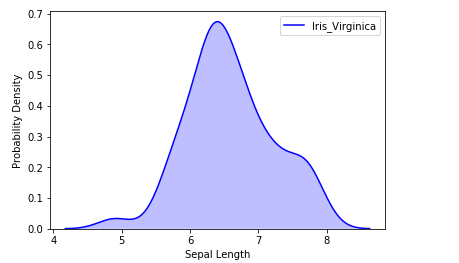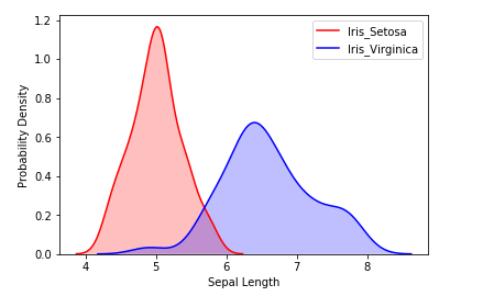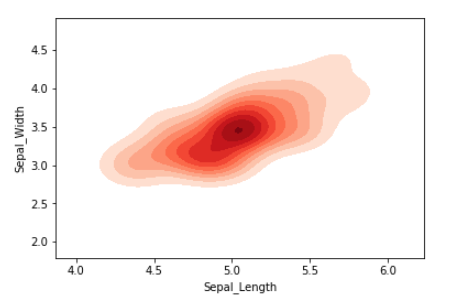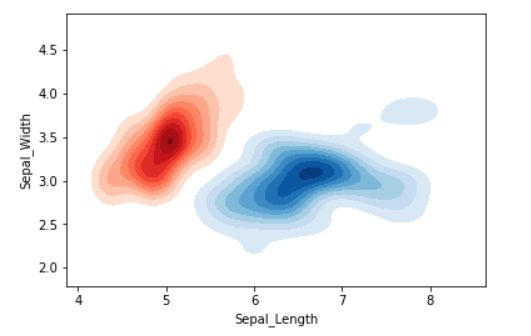Related Articles

# KDE Plot Visualization with Pandas and Seaborn

• Last Updated : 06 May, 2019

KDE Plot described as Kernel Density Estimate is used for visualizing the Probability Density of a continuous variable. It depicts the probability density at different values in a continuous variable. We can also plot a single graph for multiple samples which helps in more efficient data visualization.

In this article, we will be using Iris Dataset and KDE Plot to visualize the insights of the dataset.

1. Attributes : Petal_Length (cm), Petal_Width (cm), Sepal_Length (cm), Sepal_Width(cm)
2. Target : Iris_Virginica, Iris_Setosa, Iris_Vercicolor
3. Number of Instances : 150

One-Dimensional KDE Plot :

We can visualize the probability distribution of a sample against a single continuous attribute.

 `# importing the required libraries``from` `sklearn ``import` `datasets``import` `pandas as pd``import` `seaborn as sns``import` `matplotlib.pyplot as plt``%``matplotlib inline`` ` `# Setting up the Data Frame``iris ``=` `datasets.load_iris()`` ` `iris_df ``=` `pd.DataFrame(iris.data, columns``=``[``'Sepal_Length'``,``                      ``'Sepal_Width'``, ``'Patal_Length'``, ``'Petal_Width'``])`` ` `iris_df[``'Target'``] ``=` `iris.target`` ` `iris_df[``'Target'``].replace([``0``], ``'Iris_Setosa'``, inplace``=``True``)``iris_df[``'Target'``].replace([``1``], ``'Iris_Vercicolor'``, inplace``=``True``)``iris_df[``'Target'``].replace([``2``], ``'Iris_Virginica'``, inplace``=``True``)`` ` `# Plotting the KDE Plot``sns.kdeplot(iris_df.loc[(iris_df[``'Target'``]``=``=``'Iris_Virginica'``),``            ``'Sepal_Length'``], color``=``'b'``, shade``=``True``, Label``=``'Iris_Virginica'``)`` ` `# Setting the X and Y Label``plt.xlabel(``'Sepal Length'``)``plt.ylabel(``'Probability Density'``)`

Output:We can also visualize the probability distribution of multiple samples in a single plot.

 `# Plotting the KDE Plot``sns.kdeplot(iris_df.loc[(iris_df[``'Target'``]``=``=``'Iris_Setosa'``),``            ``'Sepal_Length'``], color``=``'r'``, shade``=``True``, Label``=``'Iris_Setosa'``)`` ` `sns.kdeplot(iris_df.loc[(iris_df[``'Target'``]``=``=``'Iris_Virginica'``), ``            ``'Sepal_Length'``], color``=``'b'``, shade``=``True``, Label``=``'Iris_Virginica'``)`` ` `plt.xlabel(``'Sepal Length'``)``plt.ylabel(``'Probability Density'``)`

Output:Two-Dimensional KDE Plot :

We can visualize the probability distribution of a sample against multiple continuous attributes.

 `# Setting up the samples``iris_setosa ``=` `iris_df.query(``"Target=='Iris_Setosa'"``)``iris_virginica ``=` `iris_df.query(``"Target=='Iris_Virginica'"``)`` ` `# Plotting the KDE Plot``sns.kdeplot(iris_setosa[``'Sepal_Length'``], ``            ``iris_setosa[``'Sepal_Width'``],``            ``color``=``'r'``, shade``=``True``, Label``=``'Iris_Setosa'``,``            ``cmap``=``"Reds"``, shade_lowest``=``False``)`

Output:We can also visualize the probability distribution of multiple samples in a single plot.

 `# Plotting the KDE Plot``sns.kdeplot(iris_setosa[``'Sepal_Length'``],``            ``iris_setosa[``'Sepal_Width'``],``            ``color``=``'r'``, shade``=``True``, Label``=``'Iris_Setosa'``,``            ``cmap``=``"Reds"``, shade_lowest``=``False``)`` ` `sns.kdeplot(iris_virginica[``'Sepal_Length'``], ``            ``iris_virginica[``'Sepal_Width'``], color``=``'b'``,``            ``shade``=``True``, Label``=``'Iris_Virginica'``,``            ``cmap``=``"Blues"``, shade_lowest``=``False``)`

Output:Attention reader! Don’t stop learning now. Get hold of all the important Machine Learning Concepts with the Machine Learning Foundation Course at a student-friendly price and become industry ready.

My Personal Notes arrow_drop_up